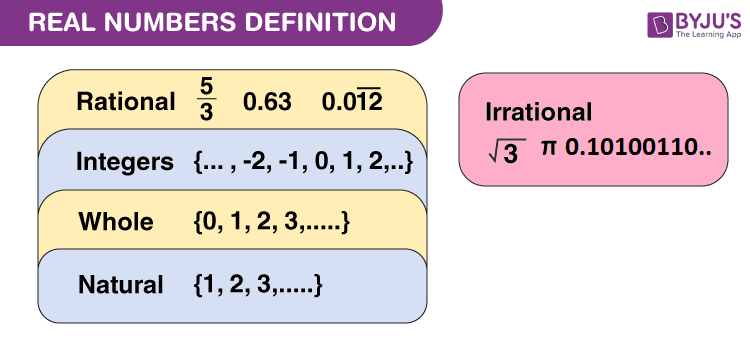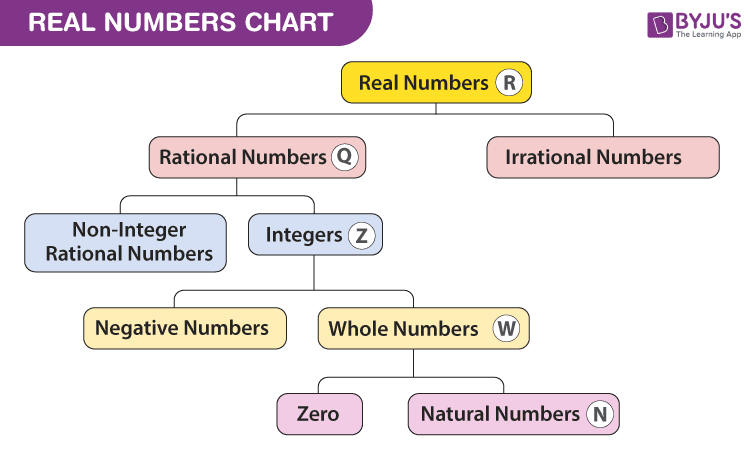# Real Numbers

Real numbers are simply the combination of rational and irrational numbers, in the number system. In general, all the arithmetic operations can be performed on these numbers and they can be represented in the number line, also. At the same time, the imaginary numbers are the un-real numbers, which cannot be expressed in the number line and are commonly used to represent a complex number. Some of the examples of real numbers are 23, -12, 6.99, 5/2, π, and so on. In this article, we are going to discuss the definition of real numbers, the properties of real numbers and the examples of real numbers with complete explanations.

## Real Numbers Definition

Real numbers can be defined as the union of both rational and irrational numbers. They can be both positive or negative and are denoted by the symbol “R”. All the natural numbers, decimals and fractions come under this category. See the figure, given below, which shows the classification of real numerals.## Set of Real Numbers

The set of real numbers consists of different categories, such as natural and whole numbers, integers, rational and irrational numbers. In the table given below, all the real numbers formulas (i.e.) the representation of the classification of real numbers are defined with examples.

Category Definition Example
Natural Numbers Contain all counting numbers which start from 1.

N = {1, 2, 3, 4,……}

All numbers such as 1, 2, 3, 4, 5, 6,…..…
Whole Numbers Collection of zero and natural numbers.

W = {0, 1, 2, 3,…..}

All numbers including 0 such as 0, 1, 2, 3, 4, 5, 6,…..…
Integers The collective result of whole numbers and negative of all natural numbers. Includes: -infinity (-∞),……..-4, -3, -2, -1, 0, 1, 2, 3, 4, ……+infinity (+∞)
Rational Numbers Numbers that can be written in the form of p/q, where q≠0. Examples of rational numbers are ½, 5/4 and 12/6 etc.
Irrational Numbers The numbers which are not rational and cannot be written in the form of p/q. Irrational numbers are non-terminating and non-repeating in nature like √2.

## Real Numbers Chart

The chart for the set of real numerals including all the types are given below:## Properties of Real Numbers

The following are the four main properties of real numbers:

• Commutative property
• Associative property
• Distributive property
• Identity property

Consider “m, n and r” are three real numbers. Then the above properties can be described using m, n, and r as shown below:

### Commutative Property

If m and n are the numbers, then the general form will be m + n = n + m for addition and m.n = n.m for multiplication.

• Addition: m + n = n + m. For example, 5 + 3 = 3 + 5, 2 + 4 = 4 + 2.
• Multiplication: m × n = n × m. For example, 5 × 3 = 3 × 5, 2 × 4 = 4 × 2.

### Associative Property

If m, n and r are the numbers. The general form will be m + (n + r) = (m + n) + r for addition(mn) r = m (nr) for multiplication.

• Addition: The general form will be m + (n + r) = (m + n) + r. An example of additive associative property is 10 + (3 + 2) = (10 + 3) + 2.
• Multiplication: (mn) r = m (nr). An example of a multiplicative associative property is (2 × 3) 4 = 2 (3 × 4).

### Distributive Property

For three numbers m, n, and r, which are real in nature, the distributive property is represented as:

m (n + r) = mn + mr and (m + n) r = mr + nr.

• Example of distributive property is: 5(2 + 3) = 5 × 2 + 5 × 3. Here, both sides will yield 25.

### Identity Property

There are additive and multiplicative identities.

• For addition: m + 0 = m. (0 is the additive identity)
• For multiplication: m × 1 = 1 × m = m. (1 is the multiplicative identity)

## Video Lesson on NumbersCommutative Property Associative Property
Distributive Property Additive Identity and Multiplicative Identity

## Example 1:

Find five rational numbers between 1/2 and 3/5.

Solution:

We shall make the denominator same for both the given rational number

(1 × 5)/(2 × 5) = 5/10  and (3 × 2)/(5 × 2) = 6/10

Now, multiply both the numerator and denominator of both the rational number by 6, we have

(5 × 6)/(10 × 6) = 30/60  and (6 × 6)/(10 × 6) = 36/60

Five rational numbers between 1/2 = 30/60 and 3/5 = 36/60 are

31/60, 32/60, 33/60, 34/60, 35/60.

Example 2:

Write the decimal equivalent of the following:

(i) 1/4  (ii) 5/8 (iii) 3/2

Solution:

(i) 1/4 = (1 × 25)/(4 × 25) = 25/100 = 0.25

(ii) 5/8 = (5 × 125)/(8 × 125) = 625/1000 = 0.625

(iii) 3/2 = (3 × 5)/(2 × 5) = 15/10 = 1.5

Example 3:

What should be multiplied to 1.25 to get the answer 1?

Solution: 1.25 = 125/100

Now if we multiply this by 100/125, we get

125/100 × 100/125 = 1

### Practice Questions

1. Which is the smallest composite number?
2. Prove that any positive odd integer is of the form 6x + 1, 6x + 3, or 6x + 5.
3. Evaluate 2 + 3 × 6 – 5.
4. What is the product of a non-zero rational number and an irrational number?
5. Can every positive integer be represented as 4x + 2 (where x is an integer)?

### Real Numbers Class 9 and 10

In real numbers Class 9, the common concepts introduced include representing real numbers on a number line, operations on real numbers, properties of real numbers, and the law of exponents for real numbers. In Class 10, some advanced concepts related to real numbers are included. Apart from what are real numbers, students will also learn about the real numbers formulas and concepts such as Euclid’s Division Lemma, Euclid’s Division Algorithm and the fundamental theorem of arithmetic in class 10.

Keep visiting BYJU’S to get more such Maths lessons in a simple, concise and easy to understand way. Also, register at BYJU’S – The Learning App to get complete assistance for Maths preparation with video lessons, notes, tips and other study materials.

## Frequently Asked Questions on Real Numbers

Q1

### What are Natural and Real Numbers?

Natural numbers are all positive integers starting from 1 to infinity. All natural numbers are integers but not all the integers are natural numbers. These are the set of all counting numbers such as 1, 2, 3, 4, 5, 6, 7, 8, 9, …….∞.

Real numbers are numbers that include both rational and irrational numbers. Rational numbers such as integers (-2, 0, 1), fractions(1/2, 2.5) and irrational numbers such as √3, π(22/7), etc., are all real numbers.

Q2

### Is Zero a Real or an Imaginary Number?

Zero is considered to be both a real and an imaginary number. As we know, imaginary numbers are the square root of non-positive real numbers. And since 0 is also a non-positive number, therefore it fulfils the criteria of the imaginary number. Whereas 0 is also a rational number, which is defined in a number line and hence a real number.

Q3

### Are there Real Numbers that are not Rational or Irrational?

No, there are no real numbers that are neither rational nor irrational. The definition of real numbers itself states that it is a combination of both rational and irrational numbers.

Q4

### Is the real number a subset of a complex number?

Yes, because a complex number is the combination of a real and imaginary number. So, if the complex number is a set then the real and imaginary numbers are the subsets of it.

Q5

### What are the properties of real numbers?

The properties of real numbers are:
Commutative Property
Associative Property
Distributive Property
Identity Property
Q6

### Is √3 a real number?

Yes, √3 is a real number. We know that a real number is a combination of both rational and irrational numbers. Since √3 is an irrational number, we can also say that √3 is a real number.

Q7

### Is 3i a real number?

No, 3i is not a real number, as it has an imaginary part in it.

Q8

### What are the different subsets of real numbers?

The subsets of real numbers include rational numbers, irrational numbers, natural numbers, and whole numbers.

Test your Knowledge on Real Numbers

1. According to a new mathematical definition, whole numbers are divided into two sets, one of which is the merger of the sequence of prime numbers and numbers zero and one. Three other definitions, deduced from this first, subdivide the set of whole numbers into four classes of numbers with own and unique arithmetic properties. The geometric distribution of these different types of whole numbers, in various closed matrices, is organized into exact value ratios to 3/2 or 1/1.

• can u use any real number of ur choice
in mathematics

2. Where is the chat box?

3. Can every positive integer be represented as 4x + 2 (where x is an integer)?